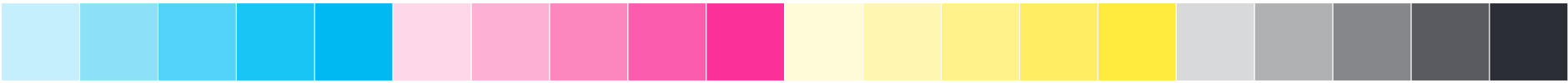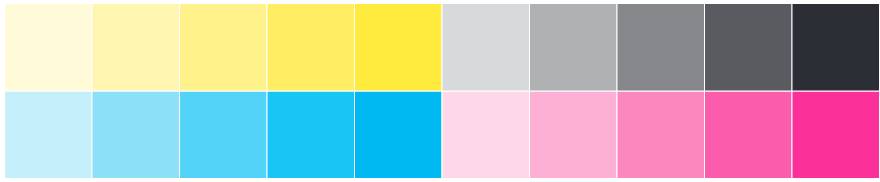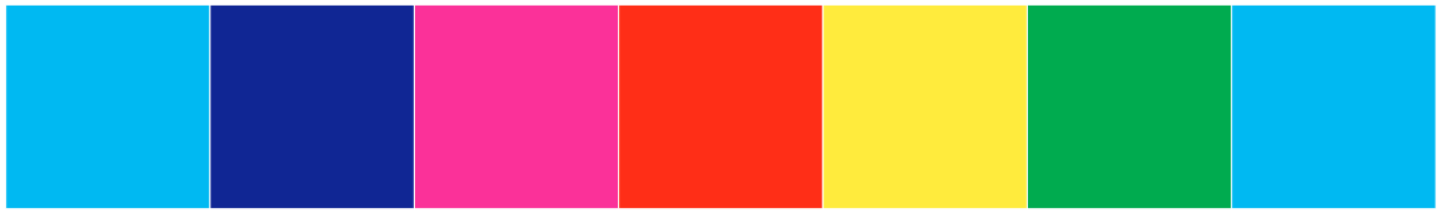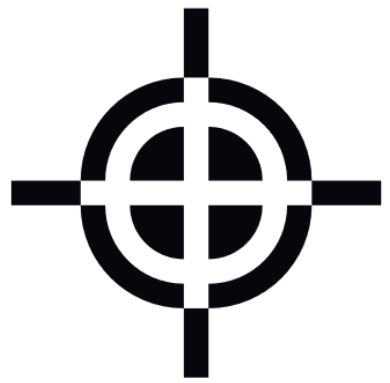# Features#

In this chapters, we will overlook some detailed algorithms and structure of implementations of each feature.

## Signature#

### Implementation#

Signature parent: Converter related class: SigComposition

It is just a simple

It is hardly interwinded with Imposition feature.

## Imposition#

Calculating layout and case of signature is not an easy task. It is a research topic in computational geometric field. However, for standard page numbers, 4, 8, 16, … 32, 64, their layouts have been well known in printing area.

The imposition is locating works of pages to paper and the result of them. Unless you bining book in old Asia style (their method can use single paper as a basic signature), you must print the signature considering fold action for efficiency. This is why the manuscript for a book should have page range which is a divisor of 4.

Since, imposition is implicitly considering fold action in post stage. Calculating its layout on single page is not an easy task.

### Standard Type#

This type signature alternately changes folding direction in each step. $$2, 4, 8, \dots, 2^n$$ leaves signatures are included in.

#### Algorithm#

It will be worth to describe folding layout update process.

• $$n$$ sheets signature contains $$n$$ number of pages.

• Imposition of $$n$$ sheets signatures are divided into two sections, the front page and the back page.

This process covers next signatures.

\begin{align}\begin{aligned}\begin{split}a_0 = 4 \\\\\end{split}\\a_n = 2 \cdot a_{n-1}\end{aligned}\end{align}

Let’s start from $$4$$ sheets signature, its page layout is a $$(1,2)$$.

Front page: $$[4, 1]$$

Back page: $$[2, 3]$$

from these two matrix, we will get page imposition of $$8$$ sheets signature.

Imagine the folding process of $$4$$ sheets signature to make $$8$$ sheets signature. We rotate 90 degrees and split them into $$2$$ sub-sections. Interesting point is that, the $$k$$-th page of $$2n$$ sheets signature always exists in $$k$$-th page of $$n$$ sheets signature, $$1 \leq k \leq n$$. In addition, the two pages, $$a, b$$, seperated by the creasing line have next relationship, $$a+b = n+1$$.

These are all we need. The remains are just following them.

##### Rotating#

Rotating elements of matrix can be divided into two steps, transpose and flip. See rotation of the elements of 90 degree in counter-clockwise direction,

Transpose:

$\begin{split}[4, 1] \rightarrow \begin{bmatrix} 4 \\ 1 \end{bmatrix}\end{split}$

Flip:

$\begin{split}\begin{bmatrix} 4 \\ 1 \end{bmatrix} \rightarrow \begin{bmatrix} 1 \\ 4 \end{bmatrix}\end{split}$
##### Expanding#

Now expand each line using $$a+b = n+1$$. Basically, in a single number case, an additional number is left of the previous number.

\begin{align}\begin{aligned}\begin{split}8 = 8 + 1 -1,  \rightarrow [8 ,1] \\\\\end{split}\\5 = 8 +1 -4,  \rightarrow [5, 4]\end{aligned}\end{align}

Then, we get a front layout matrix of the $$8$$ sheets signature. In the same way, let’s get a front layout matrix of the $$16$$ sheets signature.

Rotating:

$\begin{split}\begin{bmatrix} 8& 1 \\ 5& 4 \end{bmatrix} \rightarrow \begin{bmatrix} 1& 4 \\ 8& 5 \end{bmatrix}\end{split}$

Expanding:

Note

There is a little different in $$n>4$$ case. In expanding steps, you must divide row numbers into sub-groups whose length is $$2$$. The prior number process is the same with $$n=4$$ case but the second number is remained at right in expanding progress. For example, if we have $$[13, 12, 4, 5, 1, 8, ...]$$ row then, $$[[13, 12], [4, 5], [1, 8], ...]$$ and expand them.

$[1, 4] \rightarrow [ [16 , 1 ], [4, 13] ]$
$[8, 5] \rightarrow [ [ 9 , 8 ], [ 5 , 12 ] ]$

See update steps of front matrix:

$\begin{split}\begin{bmatrix} 4& 1\end{bmatrix} \rightarrow \begin{bmatrix} 8& 1 \\ 5& 4 \end{bmatrix} \rightarrow \begin{bmatrix} 16& 1& 4& 13 \\ 9& 8& 5& 12 \end{bmatrix}\end{split}$
##### Rotating Page#

Imposition work includes folding work. That is, pages must be rotated in the right direction to match the direction of each page after the fold. In imposition layout, it is simple. Just rotating $$2, 4, 6, ..., 2k, ...$$ rows of 180 degrees.

### Deep Type#

Deep type is applying arcodian folding in one step in fold process. This allow as more flexible layout than standard folding. For example, 12, 24 leaves imposition was impossible, but here we can acheieve right ordered page signature.

## ToImage#

Some PDF files could be broken for their page contents during the rotation of pages. To avoid such a situation, toimage routine is presented. This routine converts all pages in the manuscript to single images per page. However, it increases file size and makes converting speed slow more than 10 times.

## Printing Marker#

Printing marks are indicators of printing quality and a guide of manufacturing process.

### Registration and Color bar#

Basically, color implementation is a mixture of basis colors. In addition, printing result is hardly relying on its quality of implementation. There are various properties to determine the quality of printing.

Every colors can be represented with sum of basis colors. There are two basis in color system, RGB(Red, Green, Blue), and CMYK(Cyan, Magenta, Yellow, Key:black). RGB color system is used in light mixing and it is a basic system of nature, in color sensing of animals neuron. CMYK is a system that used in printing with mixture of inks.

RGB system is getting brighter than primary colors and CMYK is getting darker than primary colors.

Mixing mapping of colors

RGB system

• R + G + B = White

• R + G = Yellow

• R + B = Magenta

• G + B = Cyan

• None = Black

CMYK system

• C + M + Y = Registration black

• C + M = Blue

• C + Y = Green

• M + Y = Red

• K = Black

• None = White

Since, the quality of basis color printing is a charateristic property of printing device In common situation, printing devices spread inks on surface with independent spread system by colors. Therefore, if we want to check the quality of color and identify the error, we must check all basis colors.

• Dense uniformity of basis color - color bar

• Mixed color representation - color bar

• Registration of printing heads - registration marker

Various types exist in color bar for printing marker, but matrix of color square is a common type.

#### Color bar#

HornPenguin Booklet provides two types of color bars. One is for checking basis color dense and the other is for mixed coloe representation. Primary type is composed of 20%, 40%, 60%, 80%, 100% dense of each color, total 20 square. Last one is composed of 100% color mixture of CMY colors. It is a transform to 1d list of color van-diagram.

Location of color bar is determined by the pagesize of manuscript. The program chooses larger area of margin betweeen horizontal and vertical margin. Its shape is 2x10, or 1x20 matrix. In the given area, the program find a dimension that makes larger square.#### Registration Black and Marker#

It will be weird that Key color is in CMYK color, because we can make black color by mixing C, M, Y inks. However, such black does not dark as much we expected and it is a waste of inks, since the most frequently used color in printing is a black. Therefore, additional black ink is used as a name of ‘Key’. Still, CMY black is used in printing not for printing cotents but as a marker. It is called ‘registration black’. See a color vector to identify the difference.

• Black(Key): ( 0, 0, 0, 1)

• Registration Black: (1, 1, 1, 0)

In prinitng device, registration black is implemented by spread all cmyk ink on such area. It is useful to see registration of color spread system in device. Usually, cross symbol is used as registration marker. If they are not registered properly, there will be some color edges on symbol.## Note#

Note

In working

Unlikely the manuscripts for book, booklet and the others that contains its own contents, the note is designed for users to fill their own contents. Notes consist of very few template pages, even a single template. For single and very simple note template, symmetric to its center, the imposition process is not needed, but if you design a laboratory note or some complicate template, it is needed.

Some notes need page numbering, expecially laboratory note. As like note mode, you can disable or enable the numbering routine. It allows you to set next options.

• Align: Left/Center/right

• Count: Even/Odd/Both/Even only/Odd only

The count options may be confuse if you see at first. This represent the counted pages and number indicated pages.

Even: count full pages and indicate it only on even pages. Even(only): count only even pages and indicate it only on even pages.